Xi, E.-H., and Zhao, G.-J. (2011). "Research on differentiated xylem cells based on fractal dimension," BioRes. 6(3), 3066-3079.

#### Abstract

characteristics of differentiated xylem cells of the fast-growing 76’during the active phase by the method of differential box-counting fractal dimension. The fractal characteristics of differentiated xylem cells as well as the relationship between fractal dimension and tissues proportion were considered. Results showed that the fractal dimensions of cross sections were larger than those of tangential sections. Fractal dimension of cross sections had a remarkable negative correlation with the ratio of vessel element, significant positive correlations with the proportion of wood fiber and the proportion of parenchyma. The correlation of fractal dimension with wood fiber proportion was more significant than that with parenchyma proportion. The results were also verified by replacement of the tissues in the cross section. It was observed that fractal characteristics of the wood microstructure were very much related to the proportions of different tissues of the xylem cells.

RESEARCH ON DIFFERENTIATED XYLEM CELLS BASED ON FRACTAL DIMENSION

En-Hua Xi and Guang-Jie Zhao*

This study considers the fractal characteristics of differentiated xylem cells of the fast-growing Populus×euramericana cv. ‘74 /76’ during the active phase by the method of differential box-counting fractal dimension. The fractal characteristics of differentiated xylem cells as well as the relationship between fractal dimension and tissues proportion were considered. Results showed that the fractal dimensions of cross sections were larger than those of tangential sections. Fractal dimension of cross sections had a remarkable negative correlation with the ratio of vessel element, significant positive correlations with the proportion of wood fiber and the proportion of parenchyma. The correlation of fractal dimension with wood fiber proportion was more significant than that with parenchyma proportion. The results were also verified by replacement of the tissues in the cross section. It was observed that fractal characteristics of the wood microstructure were very much related to the proportions of different tissues of the xylem cells.

Keywords: Differentiated xylem cell; Populus×euram ericana cv. ‘74 /76’; Fractal characteristic; Positive correlation

Contact information: College of Materials Science and Technology, Beijing Forestry University, Beijing, China; *Corresponding author: zhaows@bjfu.edu.cn

INTRODUCTION

The complex and erratic shape description in terms of self-similarity was introduced by Mandelbrot (Mandelbrot 1977, 1982; Mandelbrot et al. 1984), who proposed the fractal geometry of nature. The conception of fractal dimension (FD) can be employed in the measurement and analysis of shape and grain. Pentland (1984, 1988) noticed that the fractal model of imaged three-dimension (3-D) surfaces can be used to obtain shape information and distinguish between smooth and rough textured regions. Rigaut (1988) used the concept for image segmentation. Peleg et al. (1984) made use of the ε-blanket method, which is a 2-D generalization of the initial approach suggested by Mandelbort (1982). Gangepain and Roques-Carmes (1986), as well as Keller et al. (1989), used variations of the box-counting (BC) approach to estimate FD. In the late 1990s, the fractal theory began to be applied in wood science research (Jose and Paulo 1997; Fan et al. 1999). Fractal analysis provides a new means (Gao et al. 2004; Fei et al. 2007) of studying structure and physical properties (Hatzikiriakos and Avramidis 1994; Jose et al. 1997; Hao and Avramidis 2001, 2003; Cao and Kamdem 2004; Tekleyohannes and Avramidis 2010) of wood. One of the first pure mathematical structures using fractal, self-similar construction was for the simulation of tree growth in so-called tree branching (Edelstein-Keshet and Ermentrout 1989; Ford et al. 1990; Niklas 1986; Prusinkiewicz and Lindenmayer 1990). Fractal analysis has been used to describe wood macroscopic texture (Wang et al. 2007; Ren et al. 2007), and results showed that the fractal dimension can characterize the roughness of wood surface. Research work has been focused not only on structural problems, but also on roughness (Strnadel et al. 2001) of fractured surfaces or fracture toughness (Morel and Valentin 1999). Konas et al. (2009) studied the correlation between impact energy and fractal dimension of wood anatomy fracture structure after impact loading (as a measure of material toughness), and proposed that wood structure included a sufficient amount of information about toughness in its original state (before the loading). However, fractal structure has not yet been fully applied on an anatomical level.

Due to seasonal changes, genetic attributes, and different effects from environ-mental factors and elements, the division process of cambium cells and formation of xylem cells can be regarded as a fractal with a time series. A random distribution of elementary parts is not usually able to form a fractal construction. Wood, as a biological material, consists of several types of cell elements. Although we can distinguish the ultrastructure of cells, the cellular elements can be characterized as individual and inde-pendent parts, which form the specific hierarchy of regular (irregular) sub-regions. Self-similar shapes of structure cannot be found on lower scales of cell structure. In contrast, the process of cell division provides lots of rules of periodical formation that can show an even better indication of cell change process and internal regularity, and therefore provides a better understanding of the complicated cell growth phenomena (Fei 1999). Thus, the BC method may provide a better way to describe wood, because it is focused on a matrix of cells where fractal structure can be expected.

The purpose of this study was to apply the fractal theory to wood anatomy, characterizing the regularity of xylem cells over time using fractal analysis, and quantitatively describing the regularity and correlation between the proportion of xylem cells and the fractal dimension of wood microstructure. The method of differential box-counting fractal analysis was applied to characterize the microstructure pictures of xylem cells in cross section and tangential section in different differentiation phases of fast-growing Populus × euramericana cv.‘74/76’, and the relationships between fractal dimension and the proportion of tissues are discussed, which will provide more informa-tion about differentiation of xylem cells.

EXPERIMENTAL

Materials

Healthy plants of fast-growing two year-old Populus×euramericana cv.‘74/76’ grown in a plantation of Xiaotangshan in Beijing’s Changping district with the same diameter at breast height (DBH) were chosen and marked. Plant materials were taken 1.3 m above the trunk and sampled once a week from April to October in 2009. On each occasion, small blocks (about 10mm ×10mm × 10mm) containing phloem, cambial zone, and xylem cells were immediately immersed in fixative formalin–acetic acid–alcohol (FAA). Upon returning to the laboratory, they were placed in the same fresh fixative under a slight vacuum for 30 min. Following vacuum, these pieces were fixed in fresh fixative and preserved at 4oC. During the sampling period, until the fifth time, sampled materials exhibited thickening secondary wall in xylem cells. For convenience, the third to twenty-third batches of test-pieces were selected for measurements.

Cross and tangential slices with a thickness of 10 μm including phloem, cambium, and xylem were cut on a sliding microtome, then stained with 1% safranine of alcohol solution for 2 hours. In the next stage, the slices were dehydrated with gradient alcohol, from 50%, 70%, 85%, 95%, 100% into xylene, and then cemented with neutral gum. After that, they were observed under an Olympus BH-2 optical microscope. According to quantitative anatomy conventional methods of wood, the proportions of vessel element, wood fiber, wood ray, and parenchyma in the xylem region near the cambium zone were measured utilizing a microscopic image analysis system. Meanwhile, the 2-D digital pictures of two sections of xylem cells in all phases were collected using a microscopic image analyzer, and then converted the original JPG format into grayscale BMP format with the size of 176.2mm × 176.2mm, horizontal, and vertical resolution of 150 dpi, bit depth of 24-bit, and width and height of 1040 × 1040 pixels. BMP images tend to be rich in image information with almost no compression. In order to avoid losing too much image texture information, pictures were set mainly in reference to the size of the image itself.

Methods

The Differential Box-Counting (DBC) method, which was first proposed by Sarlar and Chaudhuri (Sarker and Chaudhuri 1992 and 1994; Chaudhuri and Sarker 1995), is an improvement of the BC method. It contains both efficiency and reliability rolled into one. The thought of this method is that the image of size M × M pixels has been scaled down to a size s × s, where s is an integer lieing between 1 and 2 / M. The previous image was considered as a 3D space with (x, y), denoting 2D position and the third coordinate (z) denoting gray level. The (x, y) space is partitioned into grids of size s × s. On each grid there is a column of boxes of size s ×s × h. If the total number of gray levels is G, then h=s. Let the minimum and maximum gray level of the image in (i, j)th grid fall in box number k and l, respectively. Then nr (i, j) =l – k + 1 is the contributions of Nr in (i, j)th grid. Then the FD can be estimated from the least square linear fit of log (Nr) against log (1 / r).

The DBC method (Borodich 1997) is typically representative of the robust analysis of the surface objects. The investigated area is divided by a regular grid with unit length of edge according to a specified scale. The ratio of count of positive boxes (grid-cells) to count of all boxes is an indicator of fractal dimension. The DBC method has a significant advantage due to its simple evaluation of the FD in image analysis mode. In this study the fractal analysis software Fractal 3 (Ren et al. 2007), with the most common experimental techniques for fractal evaluation, was used to estimate the FD of micro-structure images of the cross sections and tangential sections.

The calculation process of FD was as follows: first of all, the input image was stored in registers Imax and Imin (where I represents the image gray value). Secondly, it was assumed = 2, then r = s / M, additionally, h was calculated through rh = r × G. In the next stage, the image map was reduced 1 / 4, then

Imax (i / 2, j / 2) = max (Imax (ij), Imax ((i + 1), j), Imax (i, (j + 1)),

Imax ((i + 1), (j + 1))),and

Imin (/ 2, j / 2) = min (Imin (ij), Imin ((i + 1), j), Imin (i, (j + 1)), Imin ((i + 1), (j + 1)));

After that, nr and Nr were calculated. In this process, s was to be doubled, step by step, until sM. Finally, the method of the least square linear was used to estimate the fractal dimension (D) of log (Nr) against log (1 / r) (Fig. 1).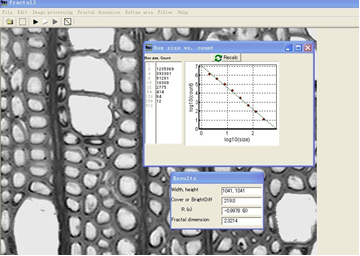Fig. 1. The calculation of fractal dimension

As illustrated in Fig. 1, the grayscale option was selected in the toolbar to calcu-late the fractal dimension of the gray image. Grayscale is the image that per pixel only has one sampling color and there are many levels of color depth between black and white. In addition, grayscale images for display usually use a nonlinear scale of each sample pixel depth to save.

RESULTS AND DISCUSSION

Fractal Analysis of Microstructure Pattern of Xylem Cells during the Active Phase

All fractal dimensions according to the mentioned method (DBC) were evaluated. In order to better analyze the results, fractal dimension curves were plotted, as shown in Figs. 2 and 3. Figure 2 shows the change of fractal dimension in cross section images during the active phase. Over the period June to September the trend was towards a sharp increase in the monthly mean fractal dimension, reaching a peak of 2.2953 in September, followed by a slight decline from September to the end of the active phase. The average value in May was approximately as great as that in June; however, that in September was roughly 1.07 times as much as that in June. Figure 2 indicates the change of fractal dimension in tangential section during the active phase. As can be seen in the figure, between May and August the average fractal dimension decreased gradually. In August, the average fractal dimension reached its lowest point of 2.1869. A gradual increase followed, but the fractal dimension leveled off at about 2.2046 from September to the end of the active phase.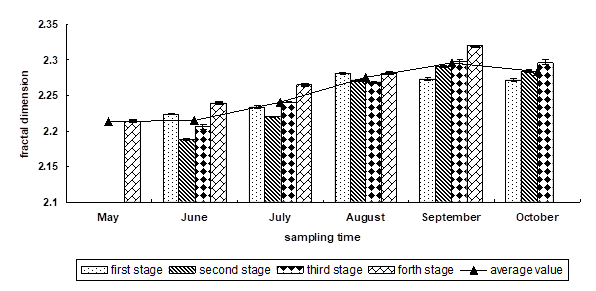A month was divided into four periods, the first stage was 7th of each month, the second one was 15th of each month, the third one was 22nd of each month, and the fourth one was 30th of each month. The following figures are the same.

Fig. 2. The distribution of fractal dimension of xylem cell images in cross section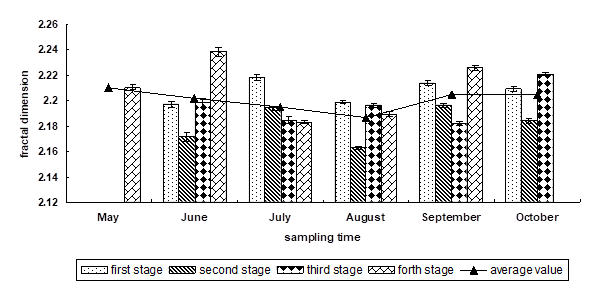Fig. 3. The distribution of fractal dimension of xylem cell images in tangential section

It also can be seen from the statistics (Table 1) that the fractal dimensions of cross sections were generally greater than those of tangential sections. This result indicates that the texture pattern composed of microstructure in cross section has a more complex degree of order. This is because the images in the cross section of wood can reflect comprehensive structural information, not only in quantity, but also in size, and they can almost characterize anatomical features of all cells. By contrast, the tangential section of wood can only reflect the length and width of cells.

Table 1. Fractal Dimensions of Microstructure Images of Xylem Cells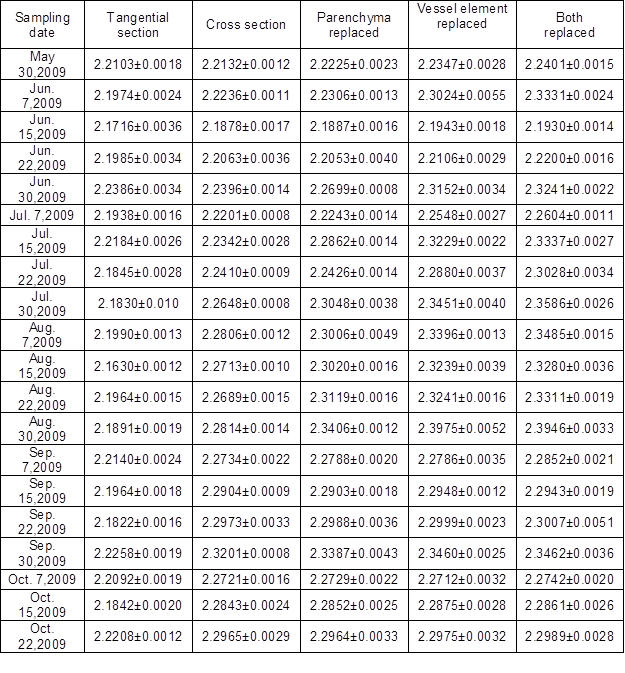The results of one-way analysis of variance (ANOA) of fractal dimension in tangential and cross section are shown in Table 2. According to the ANOVA, five kinds of fractal dimension were significantly correlated at the 0.01 level. This indicates that the fractal dimensions are largely impacted by growth time during the active phase.

Table 2. Analysis of Variance for Fractal Dimensions by Time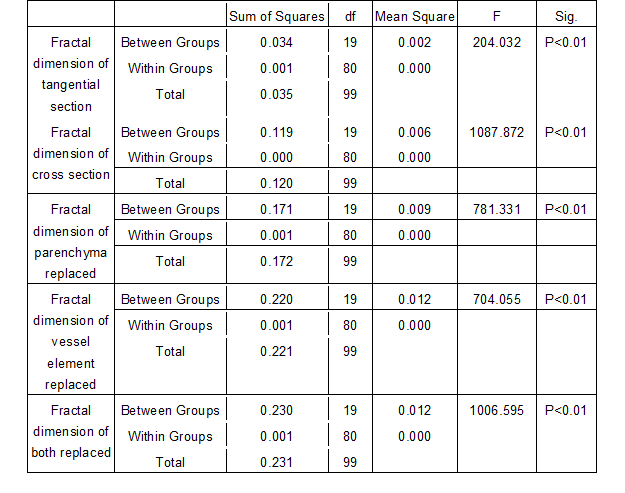Relation between Tissues Proportion of Xylem Cells and Fractal Dimension in Cross Section

Tissue proportions of xylem cells during the differentiation process were measured (Table 3). In order to better analyze the data, curves of vessel element and wood fiber proportions were plotted, and results are shown in Figs. 4 and 5. It was observed that the average proportion of vessel elements decreased gradually throughout the whole differentiation process. To be more precise, the maximum rate of decrease was 19.46% from May to June. By contrast, the proportion in June and July remained stable. Furthermore, from July to August it also had a significant reduction, and the rate was 18.97%. The rate of decline was 7.90% and 16.14% respectively from August to October. According to Fig. 4, the average proportion of wood fiber increased gradually throughout the whole process of differentiation. To be more exact, the maximum rate of increase was 12.1% from May to June. In contrast, the proportion in June and July stayed unchanged. In addition, from July to August there was another marked rise, and the growth rate was 9.50%. However, from August to October the ratio rose slightly, and the rise in rate was 3.73% and 4.46%, respectively.

Table 3. Ratio of Tissues in Differentiated Xylem Cells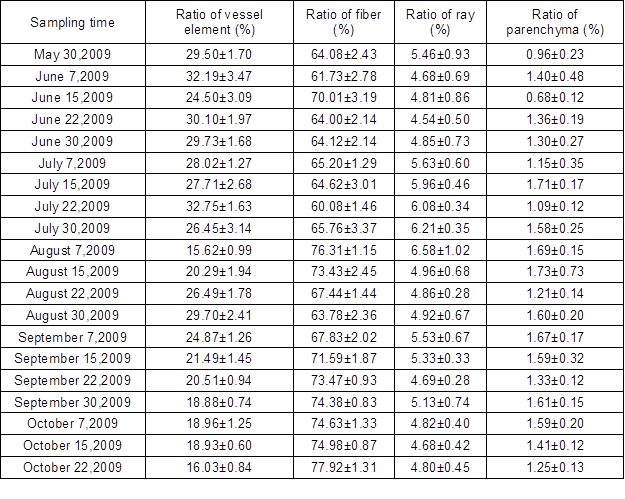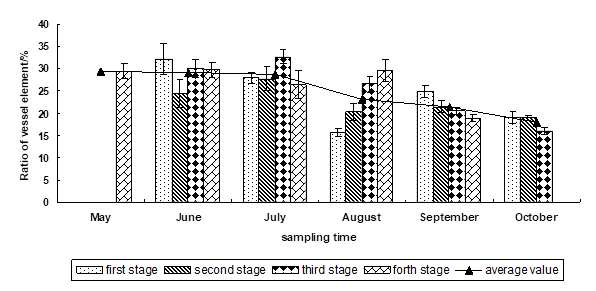Fig. 4. Changes of vessel element proportion during the differentiation process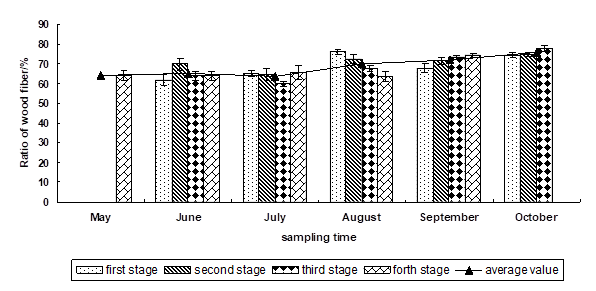Fig. 5. Changes of wood fiber proportion during the differentiation process

The results of one-way ANOA of tissue proportion are shown in Table 4. It was observed that ratio of vessel element, ratio of wood fiber, and ratio of ray and ratio of parenchyma were significantly correlated at the 0.01 level. This demonstrates that there is significant difference in tissue proportions during the active phase.

Table 4. Analysis of Variance for Tissue Proportion by Time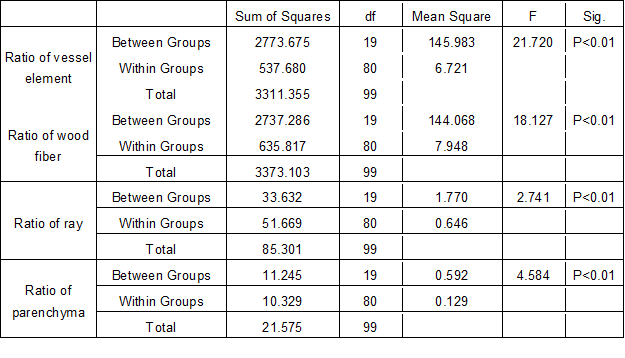Linear relationship analysis was made between tissue’s proportions and the anatomical fractal dimensions. The measured data were taken in comparison. The most significant relations are highlighted in Table 5. According to the Pearson’s analysis, the coefficient of correlation between the fractal dimensions of cross section and the ratios of vessel element, wood fiber, and parenchyma were -0.618, 0.585, and 0.354, respectively. The results showed that the fractal dimension of cross section had a highly significant negative correlation with the proportion of vessel element and a strong positive correlation with proportions of wood fiber and parenchyma cells. However, the correlation with wood fiber proportion was more notable than that with parenchyma proportion. The results also can be demonstrated from the figures. The trend of fractal dimension was opposite to that of vessel elements during the differentiation process (see Figs. 2 and 4）. It also can be seen from Figs. 2 and 5 that the trend of fractal dimension of cross section was brought into correspondence with that of wood fiber. In other words, with increasing proportion of wood fiber, the fractal dimension of cross sections increased gradually.

Table. 5. Correlation Coefficient of Fractal Dimension and Ratio of Tissues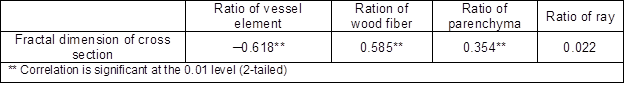In order to further verify the correlation between fractal dimensions and the proportion of tissues, some replacements were made to the cross-section images. The microstructure of xylem contains four types of cells, and the proportion of them is fixed. So, in the microstructure images, it is assumed that the proportion of one of the cells is constant, and others’ proportion is changed through replacement using the image processing software Photoshop. After that, the relationship between fractal dimension and tissues proportion can be inferred. The process of replacement is shown in Fig. 6. As the figure shows, the process had three steps. First, parenchyma was replaced by wood fiber, and then vessel element also was replaced by wood fiber. Finally, parenchyma and vessel element both were replaced by wood fiber. The fractal dimensions of replacement are listed in Table 1. In order to better analyze the results, typical months were chosen and fractal dimension curves were plotted as shown in Figs. 7 and 8. The figures compare the fractal dimension after image replacement in four stages. It was observed that the fractal dimensions of parenchyma replaced by wood fiber images were a little larger than those of the original ones. Because in that case, the ratio of vessel element was changeless, the ratio of wood fiber became larger than that of original image. The conclusion was consistent with the previous result that the fractal dimension had a more remarkable positive correlation with parenchyma than with wood fiber. However, the fractal dimensions of vessel element replaced by wood fiber images were a lot greater than those with the parenchyma replaced on account of that the ratio of vessel element was larger than that of parenchyma in the image. These findings also confirmed the earlier result that the vessel element proportion had a negative relationship with the fractal dimension. The fractal dimensions of both replaced images were the largest ones. With the increase of wood fiber in cross section images after being processed, the fractal dimensions increased correspondingly.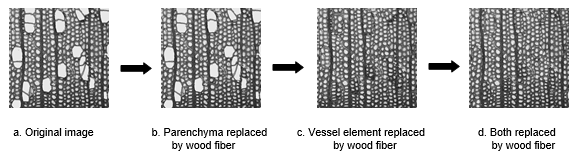Fig. 6. Replacement process of xylem cells in cross section images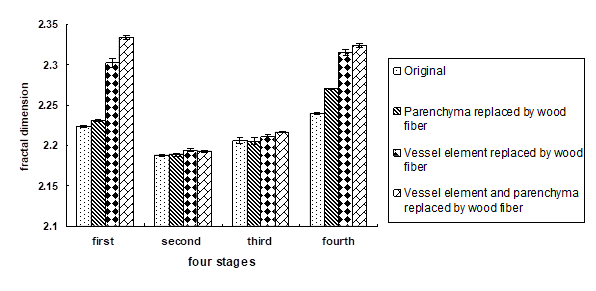Fig. 7. Changes of fractal dimensions after tissues replacement in June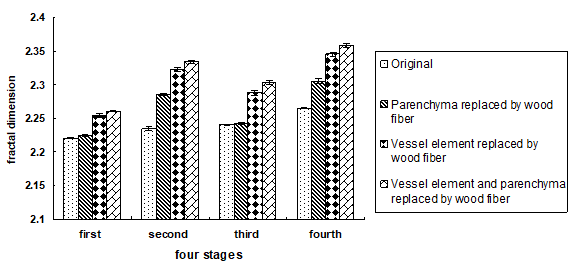Fig. 8. Changes of fractal dimensions after tissues replacement in July

The findings just described demonstrate that the changes of fractal dimension have a certain relationship with the tissues’ proportions. According to the cross section microstructure, the vessel elements account for a larger blank area being round or oval. With wood fiber cell replacement, the patterns of structure become rough and complex, so the fractal dimensions increase. Parenchyma in the cross section occupies a minor proportion of area, and it has a similar shape with wood fiber cells except for the thickness of cell wall; so after being replaced by wood fibers, the two fractal dimensions are very close. The effect of the presence of parenchyma on the fractal dimension was smaller than that of wood fiber. When both vessel elements and parenchyma tissues are substituted by wood fiber cells simultaneously, the order degree of patterns becomes more complicated and the roughness increases, so the fractal dimension increases.

This study only explored fractal dimension of cross section and tangential section. The next step should be focused on discussing the fractal dimension of three sections (including also consideration of transverse sections) and the relationship between other anatomical characteristics of xylem cells.

CONCLUSIONS

1. Fractal analysis of differentiated xylem cells demonstrated that the fractal dimension of cross sections presented a gradual increasing trend during the differentiation process of xylem cells during the active phase. By contrast, the trend of tangential section first decreased then increased.
2. In addition, the fractal dimension of cross sections was greater than that of tangential sections during the active phase, because the structural patterns of cross sections were more complicated than that of tangential sections.
3. Relations between the proportions of xylem cell tissues and fractal dimensions in cross sections during the active phase indicated that fractal dimension had a remarkable negative correlation with the ratio of vessel element, but there were significant positive correlations with the proportion of wood fiber and with the proportion of parenchyma cells. The correlation of fractal dimension with wood fiber proportion was more significant than that with parenchyma proportion. The results were also confirmed by a procedure involving tissues replacement in cross section.

ACKNOWLEDGMENTS

The authors are grateful for the support of the National Natural Science Foundation of China, Grant. No. 30871967, and the Doctoral Fund of Ministry of Education of China, Grant. No. 200800220006.

REFERENCES CITED

Borodich, F. M. (1997). “Some fractal models of fracture,” J. Mech. Phys. Solids 45(2), 239-259.

Cao, J. Z., and Kamdem, D. P. (2004). “Moisture adsorption thermodynamics of wood form fractal geometry approach,” Holzforschung 58(3), 274-279.

Chaudhuri, B. B., and Sarker, N. (1995). “Texture segmentation using fractal dimension,” J.IEEE 17(1), 72-77.

Edelstein-Keshet, L., and Ermentrout, B. (1989). “Models for branching networks in two dimensions,” SIAM J. Appl. Math. 49(4), 1136-1157.

Fan, K., Hatzikiriakos, S. G., and Avramidis, S. (1999). “Determination of the surface fractal dimension from sorption isotherms of five softwoods,” J. Wood Science and Technology 33(2), 139-149.

Fei, B. H. (1999). “Application of fractal theory on wood science,” J. China Wood Industry 13(4), 27-28.

Fei, B. H., Zhao, Y., Qin, D. C., Yang, Z., Hou, Z. Q., and Zhao, R. J. (2007). “Applying computerized tomography(CT) to study the feature of wood fracture,” J. Scientia Silvae Sinicae 43(4), 137-140.

Ford, E.D., Avery, A., and Ford, R. (1990). “Simulation of branch growth in the Pinaceae: Interaction of morphology, phenology, foliage productivity and the requirement for structural support on the export carbon,” J. Theor. Biol. 146, 1-13.

Gangenpain, J. J., and Roques-Carmes, C. (1986). “Fractal approach to two dimensional and three dimensional surface roughness,” J. Wear 109(1-4), 119-126.

##### Hao, B., and Avramidis, S. (2003). “Wood sorption fractality in the hygroscopic range. Part II. New model development and validation,” J. Wood and Fiber Sci. 35(4), 601-608.

Hatzikiriakos, S. G., and Avramidis, S. (1994). “Fractal dimension of wood surfaces from sorption isotherms,” J.Wood Science and Technology 28(4), 275-284.

Jose, A. R., and Paulo, R. C. G. (1997). “The fractal nature of wood revealed by water absorption,” J. Wood and Fiber Science 29(4), 333-339.

Keller, J. M., Crownover, R. M., and Chen, S. (1989). “Texture description and segmentation through fractal geometry,” J. Comput. Anal. of Images and Image Processing 45(2), 150-166.

Konas, P., Buchar, J., and Severa, L. (2009). “Study of correlation between the fractal dimension of wood anatomy structure and impact energy,” European Journal of Mechanics A/Solids 28,545-550.

Mandelbort, B. B. (1977). “Fractal; form, chance, and dimension,” M. San Francisco: Freeman.

Mandelbort, B. B. (1982). “The fractal geometry of nature,” M. San Francisco: Freeman.

Mandelbort, B. B., Passoja, D. E., and Paullay, A. J. (1984). “Fractal character of fracture surfaces of metals,” J. Nature 308, 721-722.

Morel, S., and Valentin, G. (1999). “Roughness of wood fractured surfaces and fracture toughness,” In: 1st RILEM Symposium on Timber Engineering, 13-15 September, Stockholm, Sweden, 171-180.

Niklas, K. J. (1986). “Computer-simulated plant evolution,” J. Sci. Am. 254, 78-86.

Peleg, S., Naor, J., Hartley, R., and Avnir, D. (1984). “Multiple resolution texture analysis and classification,” IEEE Trans. Pattern Anal. Machine Intell. 6(4), 518-523.

Pentland, A. P. (1984). “Fractal based description of nature scenes,” IEEE Trans. Pattern Anal. Machine Intell. 6(6), 661-674.

Pentland, A. P. (1986). “Shaping into texture,” J. Artificial Intell. 29, 147-170.

Prusinkiewicz, P., and Lindenmayer, A. (1990). The Algorithmic Beauty of Plants. Lecture Notes in Biomathematics, Springer, Berlin.

Redinz, J. A., and Guimaraes, P. R.C. (1997). “The fractal nature of wood revealed by water absorption,” J. Wood and Fiber Science 29(4), 333-339.

Ren, N., Yu, H. P., Liu, Y. X., and Dong, J. W. (2007). “Fractal character and calculation of wood texture (1),” J. Northeast Forestry University 35(2), 9-11.

Rigaut, J. P. (1988). “Automated image segmentation by mathematical morphology and fractal geometry,” J. Microscopy 150(1), 21-30.

Sarker, N., and Chaudhuri, B. B. (1992). “An efficient differential box-counting approach to compute fractal dimension of image,” J. Pattern Recognition 25(9), 1035-1041.

Sarker, N., and Chaudhuri, B. B. (1994). “An efficient approach to estimate fractal dimension of texture image,” IEEE Transactions on Systems, Man, and Cybernetics 24(1), 115-120.

Strnadel, B., Severa, L., and Buchar, J. (2001). “On the use of fractal analysis in description of fracture surface of wood,” Acta Universitatis Agriculturaeet Silviculturae Mendelianae Brunensis 4, 25-32.

Tekleyohannes, A. T., and Avramidis, S. (2010). “Two-level self-organization of wood properties: A new paradigm for dimensional analysis and scaling,” J. Wood Sci Technol 44(2), 253-268.

Wang, H., Wang, K. Q., Bai, X. B., and Wang, H. (2007). “The research of wood surface roughness based on fractal dimension,” J. Forest Engineering 23(2), 13-15.

Article submitted: March 24, 2011; Peer review completed: April 17, 2011; Revised version received and accepted: June 25, 2011; Published: June 28, 2011.